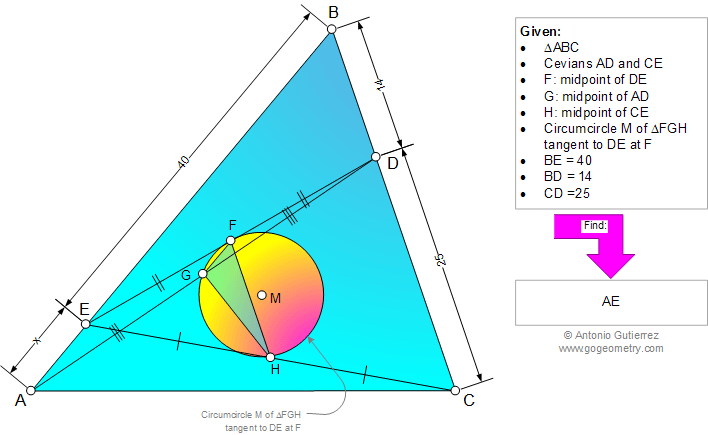# Geometry Problem 965: Triangle, Cevians, Midpoint, Circumcircle, Circle, Tangent Line, Metric Relations. Level: School, College, Mathematics Education

< PREVIOUS PROBLEM  |  NEXT PROBLEM >

 The figure shows a triangle ABC with the cevians AD and CE. F, G, and H are the midpoints of DE, AD, and CE, respectively, Circumcircle M of triangle FGH is tangent to DE at F. If BE = 40, BD = 14, and CD = 25, find AE.Home | Search | Geometry | Problems | All Problems | Open Problems | Visual Index | 10 Problems | Problems Art Gallery |  Art | 961-970 | Triangles | Midpoints | Circle | Circumcircle | Circle Tangent Line | by Antonio Gutierrez